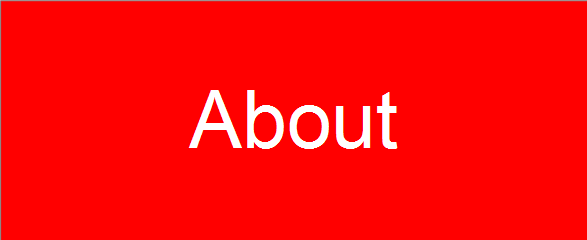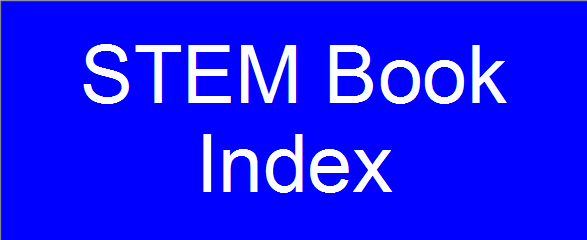• Chapter 1: Functions
• Section 1.1: Functions and Their Graphs
• Section 1.2: Combining Functions; Shifting and Scaling Graphs
• Section 1.3: Trigonometric Functions
• Section 1.4: Graphing with Software
• Section 1.5: Exponential Functions
• Section 1.6: Inverse Functions and Logarithms

• Chapter 2: Limits and Continuity
• Section 2.1: Rates of Change and Tangent Lines to Curves
• Section 2.2: Limit of a Function and Limit Laws
• Section 2.3: The Precise Definition of a Limit
• Section 2.4: One-Sided Limits
• Section 2.5: Continuity
• Section 2.6: Limits Involving Infinity; Asymptotes of Graphs

• Chapter 3: Derivatives
• Section 3.1: Tangent Lines and the Derivative at a Point
• Section 3.2: The Derivative as a Function
• Section 3.3: Differentiation Rules
• Section 3.4: The Derivative as a Rate of Change
• Section 3.5: Derivatives of Trigonometric Functions
• Section 3.6: The Chain Rule
• Section 3.7: Implicit Differentiation
• Section 3.8: Derivatives of Inverse Functions and Logarithms
• Section 3.9: Inverse Trigonometric Functions
• Section 3.10: Related Rates
• Section 3.11: Linearization and Differentials

• Chapter 4: Applications of Derivatives
• Section 4.1: Extreme Values of Functions on Closed Intervals
• Section 4.2: The Mean Value Theorem
• Section 4.3: Monotonic Functions and the First Derivative Test
• Section 4.4: Concavity and Curve Sketching
• Section 4.5: Indeterminate Forms and L'Hopital's Rule
• Section 4.6: Applied Optimization
• Section 4.7: Newton's Method
• Section 4.8: Antiderivatives

• Chapter 5: Integrals
• Section 5.1: Area and Estimating with Finite Sums
• Section 5.2: Sigma Notation and Limits of Finite Sums
• Section 5.3: The Definite Integral
• Section 5.4: The Fundamental Theorem of Calculus
• Section 5.5: Indefinite Integrals and the Substitution Method
• Section 5.6: Definite Integral Substitutions and the Area Between Curves

• Chapter 6: Applications of Definite Integrals
• Section 6.1: Volumes Using Cross-Sections
• Section 6.2: Volumes Using Cylindrical Shells
• Section 6.3: Arc Length
• Section 6.4: Areas of Surfaces of Revolution
• Section 6.5: Work and Fluid Forces
• Section 6.6: Moments and Centers of Mass
• Chapter 7: Integrals and Transcendental Functions
• Section 7.1: The Logarithm Defined as an Integral
• Section 7.2: Exponential Change and Separable Differential Equations
• Section 7.3: Hyperbolic Functions
• Section 7.4: Relative Rates of Growth

• Chapter 8: Techniques of Integration
• Section 8.1: Using Basic Integration Formulas
• Section 8.2: Integration by Parts
• Section 8.3: Trigonometric Integrals
• Section 8.4: Trigonometric Substitutions
• Section 8.5: Integration of Rational Functions by Partial Fractions
• Section 8.6: Integral Tables and Computer Algebra Systems
• Section 8.7: Numerical Integration
• Section 8.8: Improper Integrals
• Section 8.9: Probability

• Chapter 9: First-Order Differential Equations
• Section 9.1: Solutions, Slope Fields, and Euler's Method
• Section 9.2: First-Order Differential Equations
• Section 9.3: Applications
• Section 9.4: Graphical Solutions of Autonomous Equations
• Section 9.5: Systems of Equations and Phase Planes

• Chapter 10: Infinite Sequences and Series
• Section 10.1: Sequences
• Section 10.2: Infinite Series
• Section 10.3: The Integral Test
• Section 10.4: Comparison Tests
• Section 10.5: Absolute Convergence; The Ratio and Root Tests
• Section 10.6: Alternating Series and Conditional Convergence
• Section 10.7: Power Series
• Section 10.8: Taylor and Maclaurin Series
• Section 10.9: Convergence of Taylor Series
• Section 10.10: Applications of Taylor Series

• Chapter 11: Parametric Equations and Polar Coordinates
• Section 11.1: Parametrizations of Plane Curves
• Section 11.2: Calculus with Parametric Curves
• Section 11.3: Polar Coordinates
• Section 11.4: Graphing Polar Coordinate Equations
• Section 11.5: Areas and Lengths in Polar Coordinates
• Section 11.6: Conic Sections
• Section 11.7: Conics in Polar Coordinates

• Chapter 12: Vectors and the Geometry of Space
• Section 12.1: Three-Dimensional Coordinate Systems
• Section 12.2: Vectors
• Section 12.3: The Dot Product
• Section 12.4: The Cross Product
• Section 12.5: Lines and Planes in Space
• Section 12.6: Cylinders and Quadric Surfaces
• Chapter 13: Vector-Valued Functions and Motion in Space
• Section 13.1: Curves in Space and Their Tangents
• Section 13.2: Integrals of Vector Functions; Projectile Motion
• Section 13.3: Arc Length in Space
• Section 13.4: Curvature and Normal Vectors of a Curve
• Section 13.5: Tangential and Normal Components of Acceleration
• Section 13.6: Velocity and Acceleration in Polar Coordinates

• Chapter 14: Partial Derivatives
• Section 14.1: Functions of Several Variables
• Section 14.2: Limits and Continuity in Higher Dimensions
• Section 14.3: Partial Derivatives
• Section 14.4: The Chain Rule
• Section 14.5: Directional Derivatives and Gradient Vectors
• Section 14.6: Tangent Planes and Differentials
• Section 14.7: Extreme Values and Saddle Points
• Section 14.8: Lagrange Multipliers
• Section 14.9: Taylor's Formula for Two Variables
• Section 14.10: Partial Derivatives with Constrained Variables

• Chapter 15: Multiple Integrals
• Section 15.1: Double and Iterated Integrals over Rectangles
• Section 15.2: Double Integrals over General Regions
• Section 15.3: Area by Double Integration
• Section 15.4: Double Integrals in Polar Form
• Section 15.5: Triple Integrals in Rectangular Coordinates
• Section 15.6: Applications
• Section 15.7: Triple Integrals in Cylindrical and Spherical Coordinates
• Section 15.8: Substitutions in Multiple Integrals

• Chapter 16: Integrals and Vector Fields
• Section 16.1: Line Integrals of Scalar Functions
• Section 16.2: Vector Fields and Line Integrals: Work, Circulation, and Flux
• Section 16.3: Path Independence, Conservative Fields, and Potential Functions
• Section 16.4: Green's Theorem in the Plane
• Section 16.5: Surfaces and Area
• Section 16.6: Surface Integrals
• Section 16.7: Stokes' Theorem
• Section 16.8: The Divergence Theorem and a Unified Theory

• Chapter 17: Second-Order Differential Equations
• Section 17.1: Second-Order Linear Equations
• Section 17.2: Nonhomogeneous Linear Equations
• Section 17.3: Applications
• Section 17.4: Euler Equations
• Section 17.5: Power-Series Solutions

• Chapter 18: Complex Functions
• Section 18.1: Complex Numbers
• Section 18.2: Functions of a Complex Variable
• Section 18.3: Derivatives
• Section 18.4: The Cauchy-Riemann Equations
• Section 18.5: Complex Power Series
• Section 18.6: Some Complex Functions
• Section 18.7: Conformal Maps

• Chapter 19: Fourier Series and Wavelets
• Section 19.1: Periodic Functions
• Section 19.2: Summing Sines and Cosines
• Section 19.3: Vectors and Approximation in Three and More Dimensions
• Section 19.4: Approximation of Functions
• Section 19.5: Advanced Topic: The Haar System and Wavelets

Exercises 1.1.1 - 1.1.64

Exercises 1.1.65 - 1.2.52

Exercises 1.2.53 - 1.3.